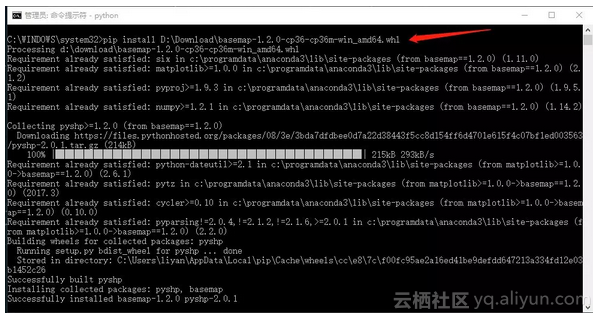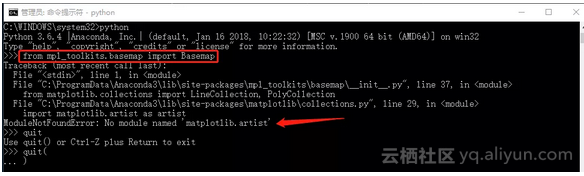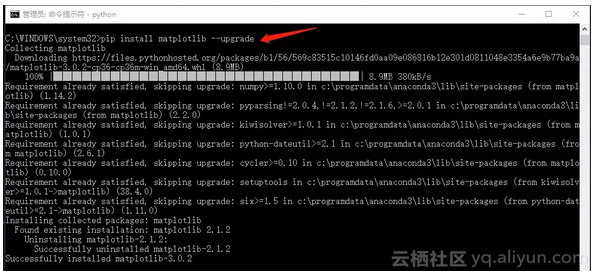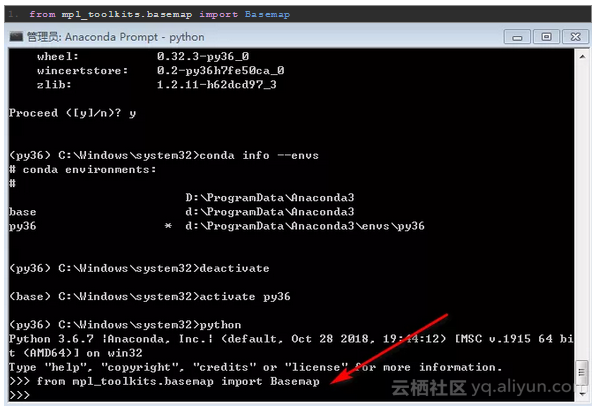#0
0
01. 云栖社区>
2. Python数据之道>
3. 博客>
4. 正文

## 历尽艰辛，Windows系统下安装Basemap，以及 PROJ_LIB 错误处理

Basemap是matplolib的一个组件，是地图数据可视化的重要工具。

# 环境一： win10

• 操作系统:Windows10

• 基于 Python3.6 版本的 Anaconda

（1）首先，从下面的网站下载 basemap 和 pyproj 的whl文件

https://www.lfd.uci.edu/~gohlke/pythonlibs/

• basemap-1.2.0-cp36-cp36m-win_amd64.whl

• pyproj-1.9.5.1-cp36-cp36m-win_amd64.whl

（2）安装 pyproj 的 whl 文件， 如下：

1. `pip install 路径+文件名`（3）安装basemap 的 whl 文件，如下：（4）测试下 basemap 是否安装成功

1. `from mpl_toolkits.basemap import Basemap`（5）更新 Matplotlib 库

1. `pip install matplotlib --upgrade`（6）再次测试 Basemap 是否安装成功1. `import matplotlib as mpl`

2. `import matplotlib.pyplot as plt`

3. `%matplotlib inline`

4. `plt.style.use('ggplot')`

5. `from mpl_toolkits.basemap import Basemap`

6. `plt.figure(figsize=(8,8))`

7. `m = Basemap(projection='ortho', resolution=None,`

8. ` lat_0=50,lon_0=-100)`

9. `m.bluemarble(scale=0.5)`# 环境二： win7

• 操作系统:Windows7

• 基于 Python3.6 版本的 Anaconda

1. `from mpl_toolkits.basemap import Basemap`

1. `Traceback (most recent call last)`

2. `<ipython-input-1-d9467465a3b6> in <module>()`

3. `----> 1 from mpl_toolkits.basemap import Basemap`

4. `/opt/conda/lib/python3.6/site-packages/mpl_toolkits/basemap/__init__.py in <module>()`

5. ` 144`

6. ` 145 # create dictionary that maps epsg codes to Basemap kwargs.`

7. `--> 146 pyproj_datadir = os.environ['PROJ_LIB']`

8. ` 147 epsgf = open(os.path.join(pyproj_datadir,'epsg'))`

9. ` 148 epsg_dict={}`

10. `/opt/conda/lib/python3.6/os.py in __getitem__(self, key)`

11. ` 667 except KeyError:`

12. ` 668 # raise KeyError with the original key value`

13. `--> 669 raise KeyError(key) from None`

14. ` 670 return self.decodevalue(value)`

15. ` 671`

16. `KeyError: 'PROJ_LIB'`

（1）在 Anaconda 下创建一个虚拟环境

1. `conda create -n py36 python=3.6`

1. `conda info --envs`

1. `activate py36`

（2）在 虚拟环境 “py36” 下安装 matplotlib

1. `pip install matplotlib`

（3）在 虚拟环境 “py36” 下安装 pyproj 的 whl 文件， 如下：

1. `pip install 路径+文件名`

（4）在 虚拟环境 “py36” 下安装basemap 的 whl 文件，如下：

1. `pip install 路径+文件名`

（5）在 虚拟环境 “py36” 下测试下 basemap 是否安装成功

1. `from mpl_toolkits.basemap import Basemap`（6）测试下在 Jupyter Notebook 中 Basemap 是否可以运行

1. `jupyter notebook`

1. `conda install nb_conda`1. `import matplotlib as mpl`

2. `import matplotlib.pyplot as plt`

3. `%matplotlib inline`

4. `plt.style.use('ggplot')`

5. `from mpl_toolkits.basemap import Basemap`

6. `plt.figure(figsize=(8,8))`

7. `m = Basemap(projection='ortho', resolution=None,`

8. ` lat_0=50,lon_0=-100)`

9. `m.bluemarble(scale=0.5)`+ 关注